# 8 2 Average Velocity Speed vs Velocity c

• Slides: 13
Download presentation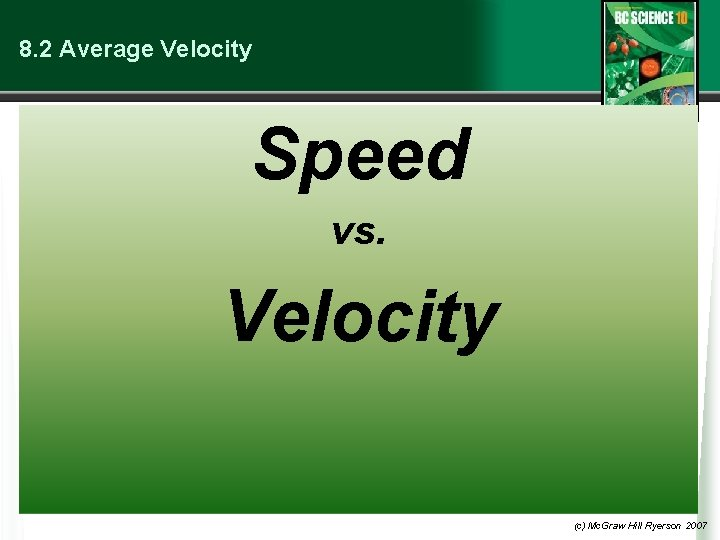8. 2 Average Velocity Speed vs. Velocity (c) Mc. Graw Hill Ryerson 2007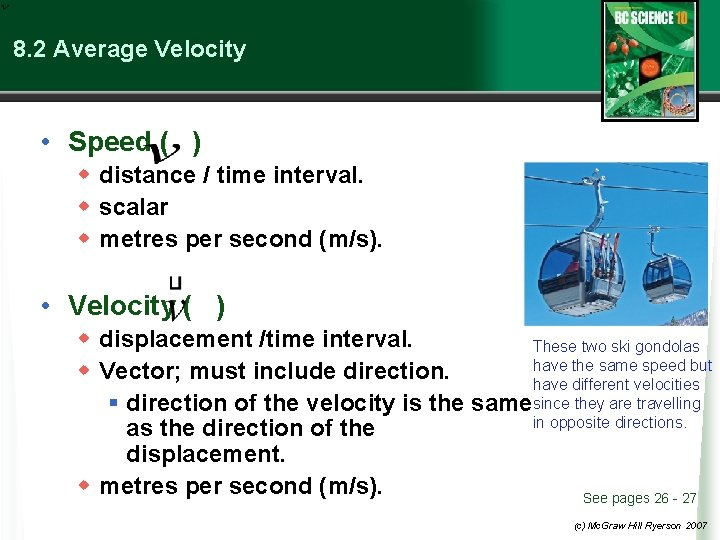8. 2 Average Velocity • Speed ( ) w distance / time interval. w scalar w metres per second (m/s). • Velocity ( ) w displacement /time interval. These two ski gondolas have the same speed but w Vector; must include direction. have different velocities § direction of the velocity is the samesince they are travelling in opposite directions. as the direction of the displacement. w metres per second (m/s). See pages 26 - 27 (c) Mc. Graw Hill Ryerson 2007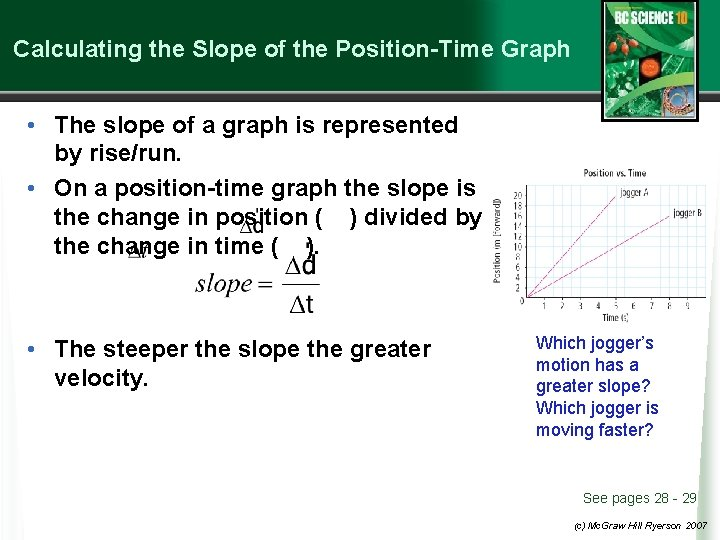Calculating the Slope of the Position-Time Graph • The slope of a graph is represented by rise/run. • On a position-time graph the slope is the change in position ( ) divided by the change in time ( ). • The steeper the slope the greater velocity. Which jogger’s motion has a greater slope? Which jogger is moving faster? See pages 28 - 29 (c) Mc. Graw Hill Ryerson 2007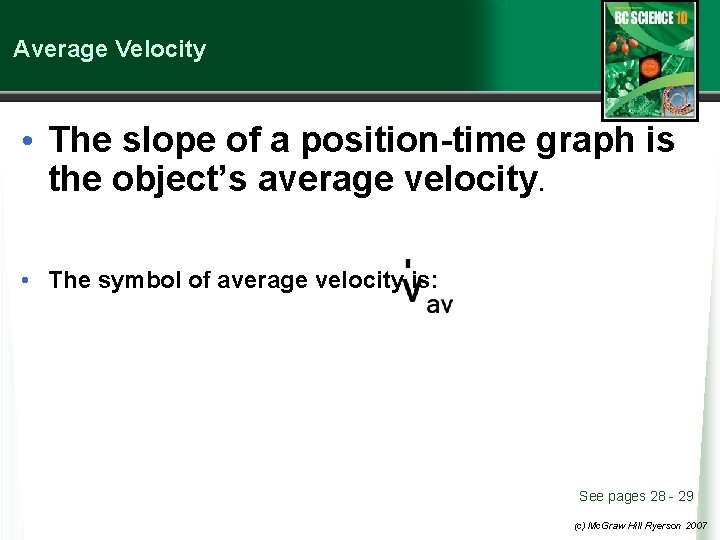Average Velocity • The slope of a position-time graph is the object’s average velocity. • The symbol of average velocity is: See pages 28 - 29 (c) Mc. Graw Hill Ryerson 2007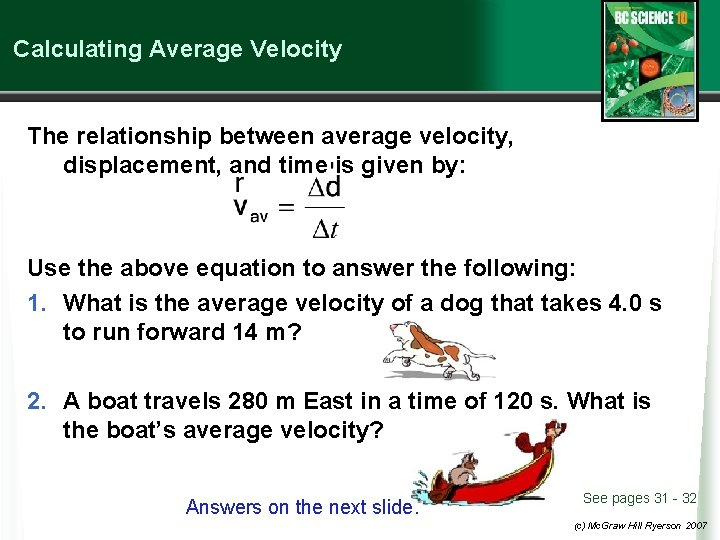Calculating Average Velocity The relationship between average velocity, displacement, and time is given by: Use the above equation to answer the following: 1. What is the average velocity of a dog that takes 4. 0 s to run forward 14 m? 2. A boat travels 280 m East in a time of 120 s. What is the boat’s average velocity? Answers on the next slide. See pages 31 - 32 (c) Mc. Graw Hill Ryerson 2007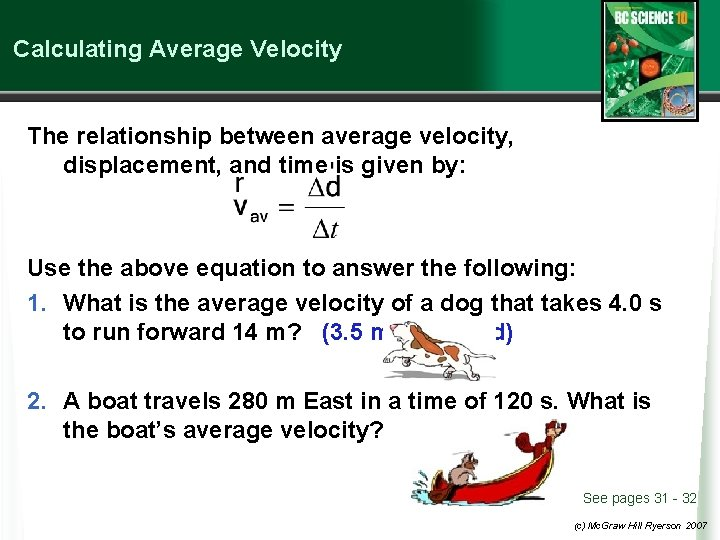Calculating Average Velocity The relationship between average velocity, displacement, and time is given by: Use the above equation to answer the following: 1. What is the average velocity of a dog that takes 4. 0 s to run forward 14 m? (3. 5 m/s forward) 2. A boat travels 280 m East in a time of 120 s. What is the boat’s average velocity? (2. 3 m/s East) See pages 31 - 32 (c) Mc. Graw Hill Ryerson 2007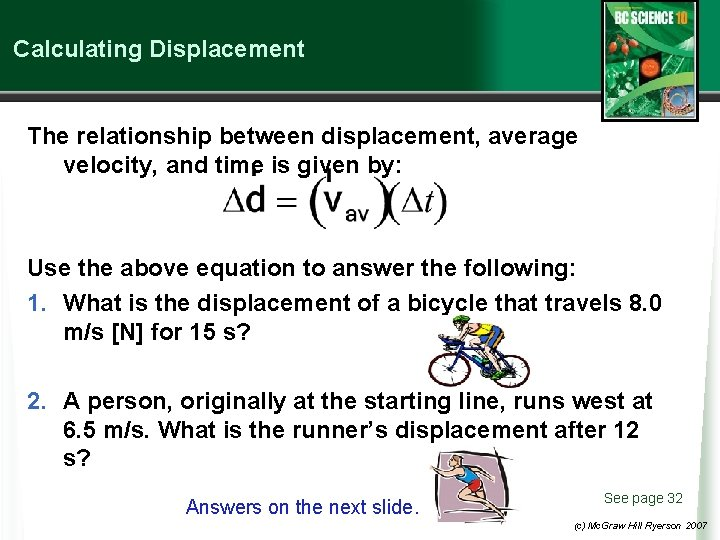Calculating Displacement The relationship between displacement, average velocity, and time is given by: Use the above equation to answer the following: 1. What is the displacement of a bicycle that travels 8. 0 m/s [N] for 15 s? 2. A person, originally at the starting line, runs west at 6. 5 m/s. What is the runner’s displacement after 12 s? Answers on the next slide. See page 32 (c) Mc. Graw Hill Ryerson 2007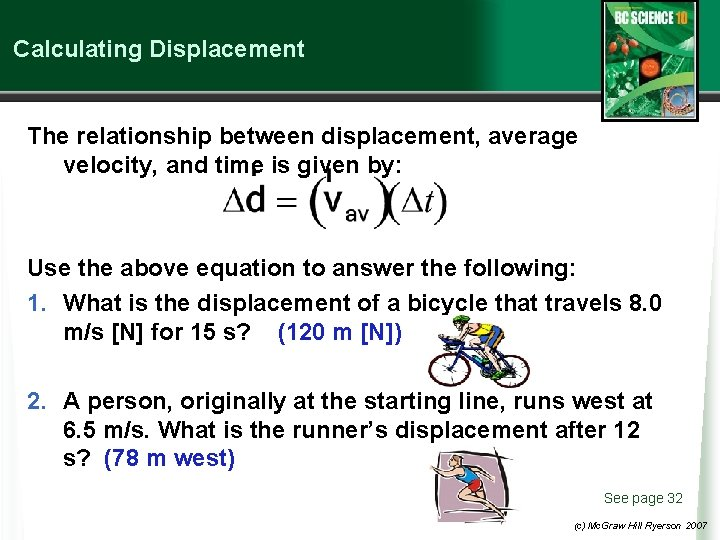Calculating Displacement The relationship between displacement, average velocity, and time is given by: Use the above equation to answer the following: 1. What is the displacement of a bicycle that travels 8. 0 m/s [N] for 15 s? (120 m [N]) 2. A person, originally at the starting line, runs west at 6. 5 m/s. What is the runner’s displacement after 12 s? (78 m west) See page 32 (c) Mc. Graw Hill Ryerson 2007Calculating Time The relationship between time, average velocity, and displacement is given by: Use the above equation to answer the following: 1. How long would it take a cat walking north at 0. 80 m/s to travel 12 m north? 2. A car is driving forward at 15 m/s. How long would it take this car to pass through an intersection that is 11 m long? See page 33 Answers on the next slide. (c) Mc. Graw Hill Ryerson 2007Calculating Time The relationship between time, average velocity, and displacement is given by: Use the above equation to answer the following: 1. How long would it take a cat walking north at 0. 80 m/s to travel 12 m north? (15 s) 2. A car is driving forward at 15 m/s. How long would it take this car to pass through an intersection that is 11 m long? (0. 73 s) See page 33 (c) Mc. Graw Hill Ryerson 2007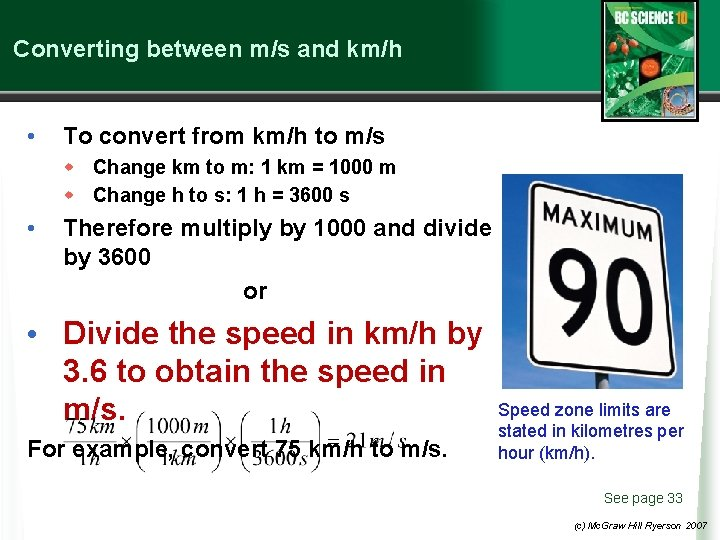Converting between m/s and km/h • To convert from km/h to m/s w Change km to m: 1 km = 1000 m w Change h to s: 1 h = 3600 s • Therefore multiply by 1000 and divide by 3600 or • Divide the speed in km/h by 3. 6 to obtain the speed in m/s. For example, convert 75 km/h to m/s. Speed zone limits are stated in kilometres per hour (km/h). See page 33 (c) Mc. Graw Hill Ryerson 2007Converting between m/s and km/h Try the following unit conversion problems yourself. 1. Convert 95 km/h to m/s. 2. A truck’s displacement is 45 km north after driving for 1. 3 hours. What was the truck’s average velocity in km/h and m/s? 3. What is the displacement of an airplane flying 480 km/h [E] during a 5. 0 min time interval? See next slide for answers See page 34 (c) Mc. Graw Hill Ryerson 2007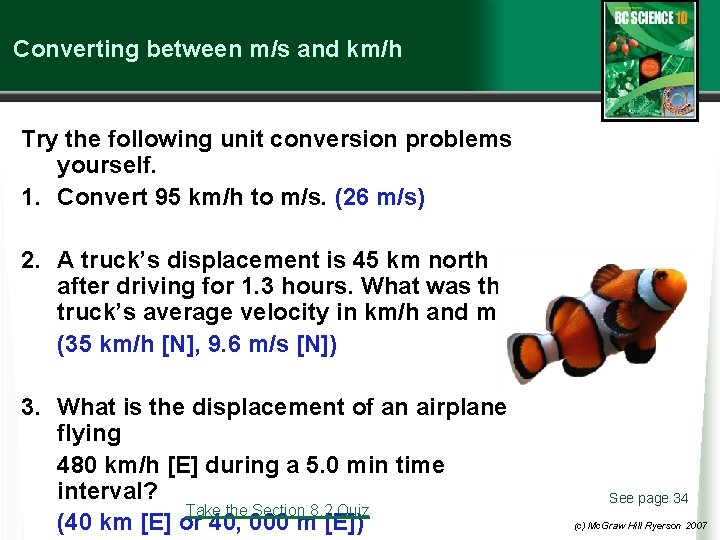Converting between m/s and km/h Try the following unit conversion problems yourself. 1. Convert 95 km/h to m/s. (26 m/s) 2. A truck’s displacement is 45 km north after driving for 1. 3 hours. What was the truck’s average velocity in km/h and m/s? (35 km/h [N], 9. 6 m/s [N]) 3. What is the displacement of an airplane flying 480 km/h [E] during a 5. 0 min time interval? Take the Section 8. 2 Quiz (40 km [E] or 40, 000 m [E]) See page 34 (c) Mc. Graw Hill Ryerson 2007